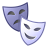# Problem in Calculate percent difference between two rasters

1049
5
09-08-2017 02:49 PMNew Contributor III

Hi,

I need to calculate the percent difference between two rasters. Below is my code and I am getting the following error. Can somebody help me to correct my code?

Error:

``````Traceback (most recent call last):
File "c:\program files (x86)\arcgis\desktop10.4\arcpy\arcpy\sa\Functions.py", line 4247, in Minus
in_raster_or_constant2)
File "c:\program files (x86)\arcgis\desktop10.4\arcpy\arcpy\sa\Utils.py", line 53, in swapper
result = wrapper(*args, **kwargs)
File "c:\program files (x86)\arcgis\desktop10.4\arcpy\arcpy\sa\Functions.py", line 4244, in Wrapper
["Minus", in_raster_or_constant1, in_raster_or_constant2])
TypeError: expected a raster or layer name‍‍‍‍‍‍‍‍‍‍‍‍‍‍‍‍‍‍‍‍``````
``````months = 
weeks = 
year = 

arcpy.env.workspace = r'H:\PRISM_800m_weekly_sum\Crawford\All'
out = r'H:\PRISM_800m_weekly_sum\Crawford\All\output'

for month in months:
for week in weeks:
arcpy.env.workspace = r'H:\PRISM_800m_weekly_sum\Crawford\All'
fmaskrasters = arcpy.ListRasters("Week_" + str(week) + "_Sum" + str(year) + "_" + str(month) + "*","*")

arcpy.env.workspace = r'H:\PRISM_800m_weekly_sum\Crawford\All\output'
normal = arcpy.ListRasters("Crawford_Normal_Week_" + str(week) + "_*_" + str(month) + "*")
Final = (outcalc2/normal)*100
finras1 = out + "\\" + "Crawford_Normal_Week_" + str(week) + "_Difference" + "_" + str(month) + ".tif"
arcpy.CopyRaster_management(Final,finras1 + "_Normal_Difference_Crawford.tif","","","","","")‍‍‍‍‍‍‍‍‍‍‍‍‍‍‍‍‍‍‍‍‍‍‍‍‍‍‍‍‍‍‍‍‍‍‍‍‍‍‍‍‍‍‍‍‍‍‍‍‍‍‍‍‍‍‍‍‍‍‍‍‍‍‍‍‍‍‍‍‍‍‍‍‍‍‍‍‍‍‍‍‍‍‍‍‍‍‍‍‍‍‍‍‍``````
Tags (3)
5 RepliesbyMVP Honored Contributor

arcpy.ListRasters() returns a list of rasters, but Minus wants two individual rasters. If you just want the first (and, presumably, only?) raster, use arcpy.ListRasters().New Contributor III

Thanks for the reply. My rasters are located in two different work spaces. I want to obtain the percent differences for the corresponding week rasters. For example,  long term weekly avarege rasters are read as normals and the weekly rasters for each year are located in fmaskrasters.   I am getting the two rasters correct in the above code but when I run Minus(normal,fmaskrasters) it gives the error mentioned above.

``````Normal Rasters:
Crawford_Normal_Week_1_1981_2016_10.tif
Crawford_Normal_Week_2_1981_2016_10.tif
Crawford_Normal_Week_3_1981_2016_10.tif
Crawford_Normal_Week_4_1981_2016_10.tif
Crawford_Normal_Week_5_1981_2016_10.tif

Weekly Rasters:
Week_1_Sum1981_10_Crawford.tif
.
.
Week_5_Sum1981_10_Crawford.tif
.
.

Week_1_Sum2010_10_Crawford.tif
Week_2_Sum2010_10_Crawford.tif
Week_3_Sum2010_10_Crawford.tif
Week_4_Sum2010_10_Crawford.tif
Week_5_Sum2010_10_Crawford.tif
.
.
Week_1_Sum2016_10_Crawford.tif
.
.
Week_5_Sum2016_10_Crawford.tif

Percent Difference = ((Week_1_Sum2010_10_Crawford.tif - Crawford_Normal_Week_1_1981_2016_10.tif) / Crawford_Normal_Week_1_1981_2016_10.tif) *100‍‍‍‍‍‍‍‍‍‍‍‍‍‍‍‍‍‍‍‍‍‍‍‍‍‍‍‍‍‍‍‍‍‍‍‍‍‍‍‍‍‍‍‍‍‍‍‍‍‍‍‍‍‍‍‍``````byMVP Honored Contributor

Well, you can only use Minus to find the difference between two rasters, not two lists of rasters, so you can either put the Minus inside a loop or somehow summarize the lists into a summary raster beforehand.New Contributor III

I am selecting one raster layer using the week, month and year defined in the main script. Therefore, "normal" and "fmaskrasters" have one raster layer. I have revised the code with what you said but still I am getting the same error.byMVP Honored Contributor